# Magnetic Circuits and Induction

## Application of Permanent Magnet Motor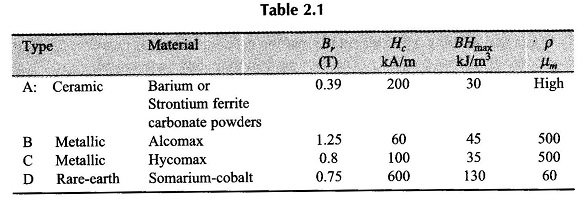Application of Permanent Magnet Motor: Application of Permanent Magnet Motor are given in Table 2.1. These properties are: residual flux density Br, coercive magnetizing force Hc maximum energy stored (BH)max and resistivity. Type A material is cheap but heavy, and suitable for low-rated production-run motors. Types B and C materials are hard and can be …

## Define Permanent Magnet in Magnetic Circuits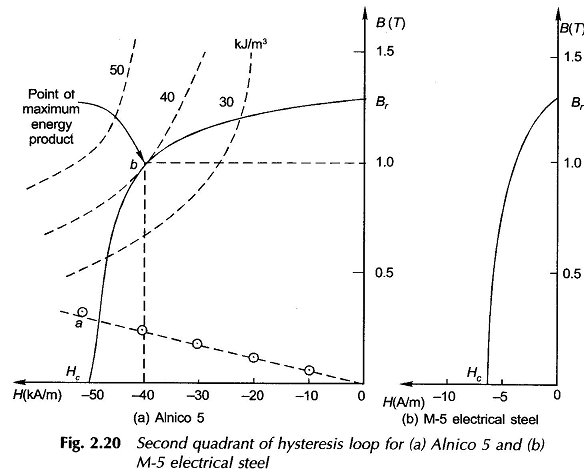Define Permanent Magnet in Magnetic Circuits: Define of Permanent Magnet is an important excitation source (life long) commonly employed for imparting energy to magnetic circuits used in rotating machines and other types of electromechanical devices. There are three classes of permanent magnet materials (or hard magnetic materials) used for permanent magnet dc (PMDC) motors: Alnicos, …

## Hysteresis Loss and Eddy Current Loss in TransformerHysteresis Loss and Eddy Current Loss in Transformer: When a magnetic material undergoes cyclic magnetization, two kinds of power losses occur in it Hysteresis Loss and Eddy Current Loss in Transformer which together are known as Core Loss in transformer. The Core Loss in transformer is important in determining heating, temperature rise, rating and efficiency …

## Energy Density Formula in Magnetic Circuit

Energy Density Formula in Magnetic Circuit: The Energy Density Formula of transformers, ac machines and several other electromagnetic devices are excited from ac rather than dc sources. With ac operation, inductance is effective even in steady-state operation. Often, the flux is determined by the impressed voltage and frequency, and the magnetization current has to adjust …

## Magnetically Induced Emf and Force

Magnetically Induced Emf and Force: Magnetically Induced Emf and Force – Faraday’s law of induction, which is the integral form of the fourth Maxwell’s equation, is given as The integrated form of Eq.(2.15) for a coil of N turns is The positive direction of current in the coil is that direction which establishes positive flux …

## Magnetic Properties of Materials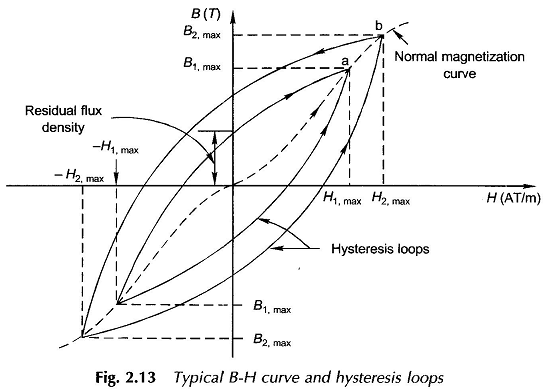Magnetic Properties of Materials: Magnetic Properties of Materials – From the magnetic point of view a material is classified according to the nature of its relative permeability (μr). All nonmagnetic materials are classified as paramagnetic ,μr slightly greater than 1, and diamagnetic, μr slightly less than 1. For all practical purposes, μr of these materials …

## Magnetic Circuit Calculations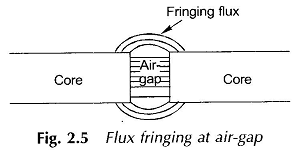Magnetic Circuit Calculations: Normally magnetic circuit calculations involve two types of problems. In the first type of problem it is required to determine the excitation (mmf) needed to establish a desired flux or flux density at a given point in a magnetic circuit. This is the normal case in designing electromechanical devices and is a …

## BH Curve Relationship of Ferromagnetic Material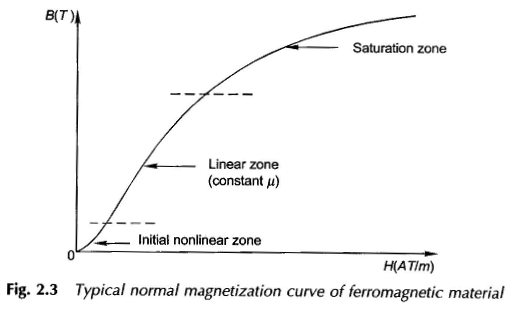BH Curve Relationship (Magnetization Characteristic) for Ferromagnetic Material: In free space (also nonmagnetic materials), the permeability μ0 is constant so that BH Curve Relationship is linear. This, however, is not the case with ferromagnetic materials used in electric machines, wherein the BH Curve Relationship of Ferromagnetic Material is strictly nonlinear in two respects saturation and hysteresis. …

## Magnetic Field Equation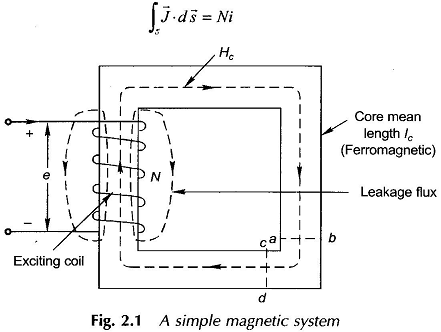Magnetic Field Equation: The exact description of the Magnetic Field Equation Basics is given by the Maxwell’s equations and the constitutive relationship of the medium in which the field is established. Such description apart from being highly complex is otherwise not necessary for use in electric machines wherein the fields (magnetic and electric) are slowly …

Scroll to Top